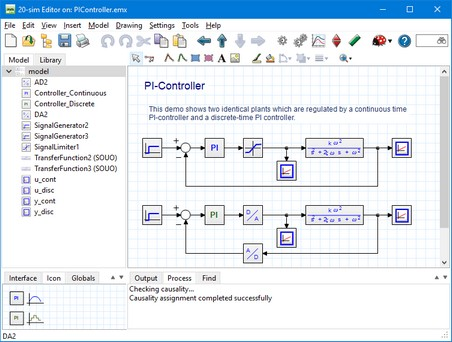﻿ 20-sim webhelp > Editor > Using Models > Continuous-Time and Discrete-Time Models

# Continuous-Time and Discrete-Time Models

In 20-sim you can model continuous-time systems, discrete-time systems and combinations of continuous-time systems and discrete-time systems (hybrid) systems. By default, models in 20-sim are continuous-time, and simulated using continuous-time integration methods. However, the program will automatically identify discrete-time parts in a model and simulate them at a fixed rate.

## Continuous-Time

Continuous-time models describe real-world processes. To simulate a continuous-time model, the model will be calculated many steps per second to get a result that is a good representation of the real-world process. If you model a car suspension system, about 100 steps per second might be sufficient to give a good representation of the behaviour of the car. If you model an electronics circuit, 100.000 steps per second might be required. The default integration method in 20-sim will automatically choose the required steps per second.

## Discrete-Time

Discrete-time models describe processes that run on computers at a fixed rate. To simulated a discrete-time model, you only have to indicate the rate at which the model should be calculated. This is called the sample rate or sample frequency. The sample rate of a discrete-time part can be set in the Simulator.

## Mixed Models

In 20-sim you can model in continuous-time and discrete-time. Mixed models containing discrete-time parts and continuous time parts are supported as well.  The example below shows a continuous time model of a PI-controlled system at the top. At the bottom the same system is shown with a discrete time controller and a continuous-time system. The discrete-time part is indicated by green input and output lines.Discrete-time parts of a model are indicated by green inputs and outputs.

Identification of Discrete-Time Parts

In 20-sim every model is continuous-time by default. Discrete-time parts of a model are identified by the occurrence of special functions:

 • sample
 • hold
 • next
 • previous
 • sampletime

Normally these functions are hidden in the equations that describe a submodel, but 20-sim will automatically recognize that the input or output of such a function should be discrete-time. If the output is discrete-time it is propagated to the next function, which output is then also "tagged" as discrete-time, and so on until the whole discrete-time part of a model is identified. A discrete time part of a model is indicated by green inputs and outputs (see previous picture).

## Connection

Continuous-time parts of a model can be connected to discrete-time parts, by using the functions:

 • sample: The sample function has a continuous-time input and a discrete-time output.
 • hold: The hold function has a discrete-time input and continuous-time output.

You can find these functions for example in the following library models:

 • DA.emx (Discrete to Analog Converter)
 • AD.emx (Analog to Digital Converter)
 • Encoder.emx (Optical Encode)

Forbidden Functions

In a discrete part of a model, certain functions are not allowed, because they are specifically meant for the use in continuous-time models:

 • algebraic
 • constraint
 • ddt
 • dly
 • event
 • eventdown
 • eventup
 • frequencyevent
 • int
 • limint
 • tdelay
 • timeevent

If you use a library model that contains such a function in a discrete-time part, an error message will be given.